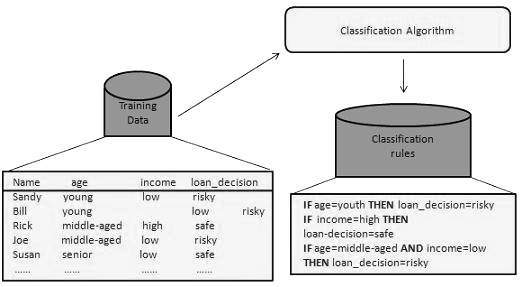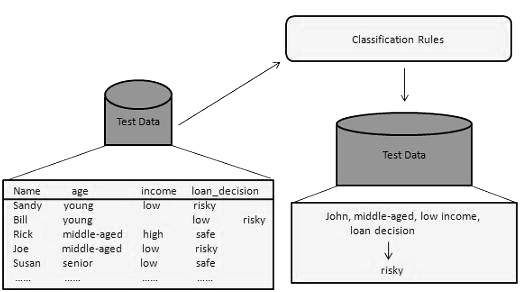# Data Mining - Classification & Prediction

There are two forms of data analysis that can be used for extracting models describing important classes or to predict future data trends. These two forms are as follows −

• Classification
• Prediction

Classification models predict categorical class labels; and prediction models predict continuous valued functions. For example, we can build a classification model to categorize bank loan applications as either safe or risky, or a prediction model to predict the expenditures in dollars of potential customers on computer equipment given their income and occupation.

## What is classification?

Following are the examples of cases where the data analysis task is Classification −

• A bank loan officer wants to analyze the data in order to know which customer (loan applicant) are risky or which are safe.

• A marketing manager at a company needs to analyze a customer with a given profile, who will buy a new computer.

In both of the above examples, a model or classifier is constructed to predict the categorical labels. These labels are risky or safe for loan application data and yes or no for marketing data.

## What is prediction?

Following are the examples of cases where the data analysis task is Prediction −

Suppose the marketing manager needs to predict how much a given customer will spend during a sale at his company. In this example we are bothered to predict a numeric value. Therefore the data analysis task is an example of numeric prediction. In this case, a model or a predictor will be constructed that predicts a continuous-valued-function or ordered value.

Note − Regression analysis is a statistical methodology that is most often used for numeric prediction.

## How Does Classification Works?

With the help of the bank loan application that we have discussed above, let us understand the working of classification. The Data Classification process includes two steps −

• Building the Classifier or Model
• Using Classifier for Classification

### Building the Classifier or Model

• This step is the learning step or the learning phase.

• In this step the classification algorithms build the classifier.

• The classifier is built from the training set made up of database tuples and their associated class labels.

• Each tuple that constitutes the training set is referred to as a category or class. These tuples can also be referred to as sample, object or data points.### Using Classifier for Classification

In this step, the classifier is used for classification. Here the test data is used to estimate the accuracy of classification rules. The classification rules can be applied to the new data tuples if the accuracy is considered acceptable.## Classification and Prediction Issues

The major issue is preparing the data for Classification and Prediction. Preparing the data involves the following activities −

• Data Cleaning − Data cleaning involves removing the noise and treatment of missing values. The noise is removed by applying smoothing techniques and the problem of missing values is solved by replacing a missing value with most commonly occurring value for that attribute.

• Relevance Analysis − Database may also have the irrelevant attributes. Correlation analysis is used to know whether any two given attributes are related.

• Data Transformation and reduction − The data can be transformed by any of the following methods.

• Normalization − The data is transformed using normalization. Normalization involves scaling all values for given attribute in order to make them fall within a small specified range. Normalization is used when in the learning step, the neural networks or the methods involving measurements are used.

• Generalization − The data can also be transformed by generalizing it to the higher concept. For this purpose we can use the concept hierarchies.

Note − Data can also be reduced by some other methods such as wavelet transformation, binning, histogram analysis, and clustering.

## Comparison of Classification and Prediction Methods

Here is the criteria for comparing the methods of Classification and Prediction −

• Accuracy − Accuracy of classifier refers to the ability of classifier. It predict the class label correctly and the accuracy of the predictor refers to how well a given predictor can guess the value of predicted attribute for a new data.

• Speed − This refers to the computational cost in generating and using the classifier or predictor.

• Robustness − It refers to the ability of classifier or predictor to make correct predictions from given noisy data.

• Scalability − Scalability refers to the ability to construct the classifier or predictor efficiently; given large amount of data.

• Interpretability − It refers to what extent the classifier or predictor understands.